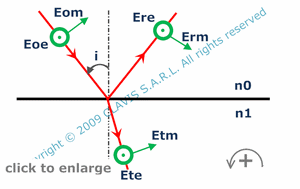and orphysical optics
polarization

# reflection and transmission amplitude ratios by a plane refracting surface

Caution
Fill in all input cells.
Choose all parameters unit.
n0 and n1 can be either values or references of glasses from the catalog.
If values only entered, the wavelength will not be considered.
A negative result means that there is a phase shift of 180° between the incident wave and the reflected-transmitted wave.
Parameters are algebric.
Angle positive way is counterclockwise according the round arrow in the bottom right of the scheme.

InputValueunit
n0
n1
i
OutputValueUnit
rmto be calculated
tmto be calculated
reto be calculated
teto be calculatedn0 : refraction index of the first medium n1 : refraction index of the second medium rm : reflection coefficient for TM polarization
( rm = Erm / Eom )
tm : transmission coefficient for TM polarization
( tm = Etm / Eom )
re : reflection coefficient for TE polarization
( re = Ere / Eoe )
te : transmission coefficient for TE polarization
( te = Ete / Eoe )

Note
This calculation page can not detect all unrealistic inputs.